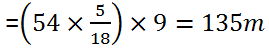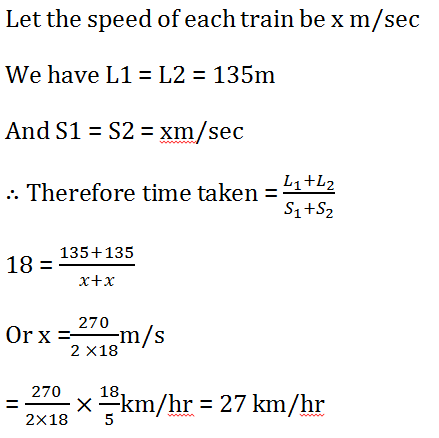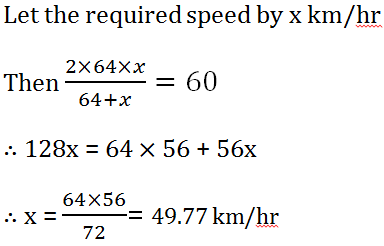New Students Offer - Use Code HELLO

# Time and Distance Problems with Solutions

Time and Distance is an important chapter of quantitative aptitude. In every numerical ability exam, at least one question can be seen related to this chapter. Before solving questions below read Time and Distance concepts.

## Ques 1.

How many minutes Raman will take to cover a distance of 400 meters if he runs at a speed of 20 km/hr ?
a. 2 mins
b. 1.5 mins
c. 1⅕ mins
d. 2.5 mins
e. None of these
Explanation :
⇒ Raman's speed = 20 km/hr = 20 × 5/18 = 50/9 m/sec
⇒ 400 × 9/50 = 1⅕ mins

## Ques 2.

John travelled from his town to city.  John went to city by bicycle at the speed of 25 km/h and came back at the speed of 4 km/h. If John took 5 hours and 48 min to complete his journey, what is the distance between town and city ?
a. 15 km
b. 22 km
c. 20 km
d. 25 km

Explanation :
⇒ Average speed of John = 2xy/x+y =  2 × 25 × 4 / 25 + 4= 200/29 km/h
⇒ Distance traveled = Speed × Time = 200/29 × 29/5 = 40 Km
⇒ Distance between city and town = 40/2 = 20 km

## Ques 3.

Speed of a train is 20 meters per second. It can cross a pole in 10 seconds. What is the length of train ?
a. 150 m
b. 250 m
c. 200 m
d. 300 m

Explanation :
⇒ Lenght of train = 20 × 10 = 200 meters

## Ques 4.

Ram walks at a speed of  12 km/h. Today the day was very hot so walked at ⅚ of his average speed. He arrived his school 10 minutes late. Find the usual time he takes to cover the distance between his school and home?
a. 40 mins
b. 45 mins
c. 50 mins
d. 60 mins

Explanation :
⇒ If Ram is walking at ⅚  of his usual speed that means he is taking 6/5 of using time.
⇒ 6/5 of usual time - usual time = 10 mins
⇒ 1/5 of usual time = 10 mins
⇒ Usual time = 50 mins

## Ques 5.

A car running at 65 km/h takes one hour to cover a distance. If the speed is reduced by 15 km/hour then in how much time it will cover the distance  ?
a. 72 mins
b. 78 mins
c. 76 mins
d. None of these

Explanation :
⇒Reduced speed = 65-15 = 50 km/h
⇒ Now car will take 65/50 × 60 mins = 78 mins

## Ques 6.

In a 100 m race A runs at a speed of 1.66 m/s. If A gives a start of 4m to B and still beats him by 12 seconds. What is the speed of B  ?
a. 1 m/s
b. 1.33 m/s
c. 1.25 m/s
d. 1.5 m/s

Explanation :
⇒Time taken by A to cover 100 meters = 60 seconds
⇒ Since A gives a start of 4 seconds then time takes by B = 72 seconds
⇒ B takes 72 seconds to cover 96 meters
⇒ Speed of B = 96/72 = 1.33 m/s

## Ques 7.

In a kilometer race, A beats B by 100 meters. B beats C by 100 meters. By how much meters does A beat C in the same race ?
a. 200 meters
b. 180 meters
c. 190 meters
d. 210 meters

Explanation :
⇒ While A covers 1000 meters, B can cover 900 meters
⇒ While B covers 1000 meters, C can cover 900 meters
⇒ Lets assume that all three of them are running same race. So when B runs 900 meters, C can run 900 × 9/10 =810
⇒ So A can beat C by 190 meters.

## Question 8.

Without any stoppage a person travels a certain distance at an average speed of 42 km/hr and with stoppages he covers the same distance at an average speed of 28 km/hr. How many minutes per hour does he stop?
(a) 25 minutes
(b) 30 minutes
(c) 20 minutes
(d) None of these

Ans 8. (C)## Question 9.

A train passes through a telegraph post in 9 seconds moving with a speed of 54 km per hour. The length of the train is
(a) 135 metres
(c) 125 metres
(b) 145 metres
(d) None of these

Ans 9. (a)
Length of the train = Speed of the train✕ Time taken in crossing the post## Question 10.

A train 50 m long passes a platform 100 m long in 10 seconds. The speed of the train in m/sec. is
(a) 25
(b) 35
(c) 15
(d) None of these

Ans 10. (b)## Question 11.

A train 300 metres long is running at a speed of 90 km/hr. How many seconds will it take to cross a 200 metres long train running in the same direction at a speed of 60 km/hr?
(a) 70 sec
(b) 50 sec
(c) 60 sec
(d) None of these

Ans 11. (b)
Here L1 = 300m, L2= 200m
S1 = 90 km/hr and S2= 60 km/hr
S1 = S2 = 90 - 60 = 30 km/hr = 30 ✕ 5/18 m/s## Question 12.

Two trains are running in opposite directions with the same speed. If the length of each train is 135 metres and they cross each other in 18 seconds, the speed of each train is
(a) 29 km/hr
(b) 35 km/hr
(c) 27 km/hr
(d) None of these

Ans 12. (c)## Question 13.

A and B are two stations. A train goes from A to B A at 64 km/hr and returns to A at a slower speed. If its average speed for the whole journey is 56 km/hr, at what speed did it return?
(a) 48 km/hr
(b) 49.77 km/hr
(c) 30 km/hr
(d) 47.46 km/hr

Ans 13. (b)## Question 14.

Excluding stoppages, the speed of a bus is 54 km/hr and including stoppages, it is 45 km/hr. For how many minutes does the bus stop per hour?
(a) 9
(b) 10
(c) 12
(d) 20

Ans 14. (b)
Due to stoppages, it covers 9km less per hour.
Time is taken to cover 9 km = (9/54×60)min
= 10 min
So, the bus stops for 10 min. per hr.

## Question 15.

Ramesh sees a train passing over 1 km long bridge. The length of the train is half that of bridge. If the train clears the bridge in 2 minutes, the speed of the train is
(a) 45 km/hr
(b) 43 km/hr
(c)50 km/hr
(d) None of these

Ans 15. (a)## Question 16.

Amit started cycling along the boundaries of a square field from cover point A. After half an hour he reached the corner point C, diagonally opposite to A. if his speed was 8 km/hr, what is the area of the field in square km
(a) 64
(b) 8
(c) 4
(d) cannot be determined

Ans 16. (c)

## Question 17.

A train 110 metres in length passes a man walking at the speed of 6 km/hr. against it in 6 seconds. The speed of the train in km per hour is
(a) 60 km/hr
(b) 45 km/hr
(c) 50 km/hr
(d) 55 km/hr